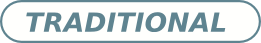# Beltrami flow structure in a diffuser. Quasi-cylindrical approximation

## Authors

• Rafael González Universidad Nacional de General Sarmiento
• Ricardo Page Universidad Nacional de General Sarmiento

## Keywords:

Swirling Flow, Beltrami, Kelvin Waves, Vortex Breakdown

## Abstract

We determine the flow structure in an axisymmetric diffuser or expansion region connecting two cylindrical pipes when the inlet flow is a solid body rotation with a uniform axial flow of speeds $\Omega$ and U, respectively. A quasi-cylindrical approximation is made in order to solve the steady Euler equation, mainly the Bragg-Hawthorne equation. As in our previous work on the cylindrical region downstream [R González et al., Phys. Fluids 20, 24106 (2008); R. González et al., Phys. Fluids 22, 74102 (2010), R González et al., J. Phys.: Conf. Ser. 296, 012024 (2011)], the steady flow in the transition region shows a Beltrami flow structure. The Beltrami flow is defined as a field $v_B$ that satisfies $\omega_B=\nabla \times v_B= \gamma v_B$, with $\gamma=constant$. We say that the flow has a Beltrami flow structure when it can be put in the form $v = U e_z + \Omega r e_{\theta} + v_B$, being U and $\Omega$ constants, i.e it is the superposition of a solid body rotation and translation with a Beltrami one. Therefore, those findings about flow stability hold. The quasi-cylindrical solutions do not branch off and the results do not depend on the chosen transition profile in view of the boundary conditions considered. By comparing this with our earliest work, we relate the critical Rossby number $\vartheta_{cs}$ (stagnation) to the corresponding one at the fold $\vartheta_{cf}$ [J. D. Buntine et al., Proc. R. Soc. Lond. A 449, 139 (1995)].

Received: 29 August 2011,, Accepted: 29 February 2012; Edited by: J-P. Hulin; DOI: http://dx.doi.org/10.4279/PIP.040002

Cite as: R González, R Page, A S Sartarelli, Papers in Physics 4, 040002 (2012)2012-03-07

## How to Cite

González, R., Page, R., & Sartarelli, A. S. (2012). Beltrami flow structure in a diffuser. Quasi-cylindrical approximation. Papers in Physics, 4, 040002. https://doi.org/10.4279/pip.040002

Articles